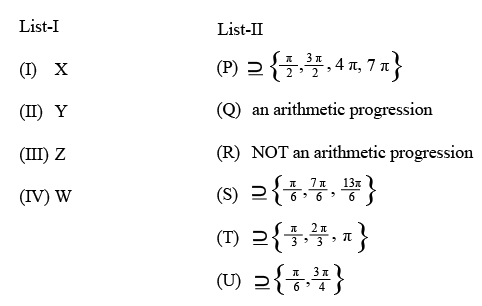### JEE (Advanced) 2019 Paper-2 Question 52

Instructions

Answer by appropriately matching the lists based on the information given in the paragraph.
Let $$f(x) = \sin (\pi \cos x)$$ and $$g(x) = \cos (2\pi \sin x)$$ be two functions defined for $$x > 0.$$ Define the following sets whose elements are written in the increasing order:
$$X = {x : f(x) = 0}, Y = {x : f'(x) = 0},$$
$$Z = {x : g(x) = 0}, W = {x : g'(x) = 0}.$$
List —I contains the sets X, Y, Z and W. List —II contains some information regarding these sets.Question 52